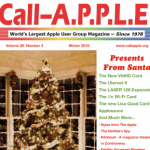# Rounding in BASIC

by Graeme Philipson
Australian Apple Review March 1984

BASIC is in many ways a great computer language. It is very easy to learn, in fact it was originally designed as a language to teach people how to program. The letters “B.A.S.I.C.” stand for “Beginners All-purpose Symbolic Instruction Code”. It is also suited to a wide range of applications: just about any programming job can be written in BASIC. Many other languages are much better suited for particular jobs, but few match BASlC’s flexibility. It is not surprising that it has become the most common language for microcomputers (and therefore for computers in general~ because there are far more micros than anything else).

But BASIC has many disadvantages. It is comparatively cumbersome, and it is “unstructured”: ie it lacks any internal order. Ask a dozen people to write a program in BASIC and they’ll write it a dozen different ways. It makes it very hard to understand other people’s programs. Whatever BASlC’s pros and cons, we are stuck with it. It is the first language learnt by virtually all Apple owners, if only because it is built into the machine. Once the novice programmer gets beyond “PRINT” statements and learning how to perform simple calculations, problems start occurring, many of them because of the language’s inherent weaknesses. A very common such problem is that of number rounding.

Round and round she doesn’t go

When calculations are performed on numbers in BASIC, the results are calculated to as many decimal places as necessary, up to 8. For example, if you divide 4.5 by 3.7 you get the answer 1.21621622. Both divisor and quotient have one decimal place, but your answer has 8. This is often not a problem. For mathematical calculations you want all the accuracy you can get. But it can bea little untidy when calculating monetary amounts, particularly if you want to print your results in nice columns, every amount with two numbers after the decimal point. That can be tricky but it is still not that difficult.

Rounding to integers Rounding numbers so that they don’t contain too many decimal points is very easy. Applesoft BASIC, like most BASlCs, contains an INT function which rounds numbers down to the number below. For example:

I NT 5.46 returns the value “5”. The trouble is,

I NT 5.999 also returns the value “5”.

What you need to do is add .5 to each number before you find its integral value. This will then return the integer CLOSEST TO the original number rather than the integer BELOW the original number. The form of the expression is X = I NT (Y + .5) where “X” is the rounded number and “Y” is the number you want rounded.

So far so good. This is still basic BASIC. Things become a little more difficult when you want to round numbers to a certain number of decimal places, for example h~o, or six. In this case we have to do a little juggling, by adding a smaller figure and multiplying and dividing. To get a number to round to two decimal places we use the expression X = INT ((Y + .005) * 100) / 100

Similarly, to round to three decimal places use the expression X = I NT ((Y + .0005) * 1000) / 1000

Unwanted fractions

Sometimes when performing calculations in Applesoft BASIC you will get small fractions on the ends of numbers which should not be there. A calculation to which you know the answer to be “5” might return the value “5.000001” or “4.99999999”. This is because of the method computers use to calculate: they use binary numbers, we use decimal. When a computer converts two numbers to binary, then performs calculations on them, then converts them back to decimal, it is possible that small discrepancies may occur through the compounding of almost infinitesimally small errors brought about by inexact binary-decimal conversions. Using the above rounding procedures will get rid of this problem .